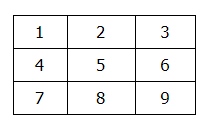# Python Tic Tac Toe Game

In this tutorial we are going to see how we can implement the tic tac toe game in Python. We can either make use of random numbers for the computer move or we can develop a simple algorithm which will play the role of a computer.

Let us first see the representation of our board. For the purpose of this tutorial every place in the board is given a number. The board will look like this.So when we say the 9th position is being assigned an X it means the last box of the board will be assigned an X.

Using Random Numbers:

Here we can keep a list of blank spaces on the board. Everytime when it’s computer’s turn we will generate a random number between [0,size_of_list-1] and computer will play that the move on the number present on that index. Here is the code-snippet for the computer move using a random number generator.

Here empty_place will store all the positions on the board which are empty and then we will take a random positions from the list and make sure that the computer’s move.

Developing Computer Algorithm:

The algorithm will consist of five steps:

1. First we will see if there is a move that computer can make which will win the game. If there is so, take that move. Otherwise, go to step 2.
2. See if there is a move that player can make which will cause computer to lose game. If there is so, move there to block the player. Otherwise, go to step 3.
3. Check if any of the corner spaces (spaces 1, 3, 7, or 9) are free. If so, move there. If no corner piece is free, then go to step 4.
4. Check if the center is free or not. If so, move there. If it isn’t, then go to step 5.
5. Move on any of the side pieces (spaces 2, 4, 6, or 8). Now there are no more steps, because if execution reaches step 5 the side spaces are the only spaces left.

Our simple algorithm is now ready. Let us first complete other requirements and then we will see the code for this algorithm.

We will need to display the board after every turn.

For the board we will take a list of size 9 which will contain the position at the start and later they will be changed to X and O.

At the start of the game, we first need to decide player will get X or O and whose turn will be first. This can be either done automatically using random number or we can take input from user. For this tutorial we are doing it using random numbers.

We will then create a new board (i.e A list having values from 1 to 9).

To check if move is valid:

Everytime we are making a move we should keep in mind that the position should not be already occupied (If a position contain the X or O then its occupied otherwise it will contain position number).

To check if player has won:

To check if a player won we have to check 2 diagonal entries, 3 rows and 3 columns. We will make a list of all the winning combinations and go through each after every turn of player or computer to check if they won.

The winning combinations are as below:

Row-wise: (0,1,2), (3,4,5), (6,7,8)

Column-wise: (0,3,6), (1,4,7), (2,5,8)

Diagonal-wise: (0,4,8), (2,4,6)

To check next move of computer:

If in a turn computer cannot win and we aren’t blocking the players pieces then we have to decide what move computer will play (i.e. If we are at step 3 of algorithm). Now according to the preference of our algorithm we should first check corner places, then center and then the rest. So we will create a list which will store these steps in the correct order and we will go through this list and make our move.

Corner: (1,3,7,9)

Center: (5)

Others: (2,4,6,8).

Now let us see how the code looks :

## Python Tic Tac Toe Game Code

Output:

Player is [X] and computer is [O]
Computer will play first.
| |
———-
| |
———-
| |
———-

O | |
———-
| |
———-
| |
———-
Make your move [1-9] : 2
O | X |
———-
| |
———-
O | |
———-
Make your move [1-9] : 4
O | X | O
———-
X | |
———-
O | |
———-
Make your move [1-9] : 5
O | X | O
———-
X | X | O
———-
O | |
———-
Make your move [1-9] : 8
O | X | O
———-
X | X | O
———-
O | X |
———-
You won !!

In the replace computer_move with random_move in the function calling then the computer will take random moves instead of using the algorithm as discussed before.

Commet down below if you have any queries related to python tic tac toe game implementation.